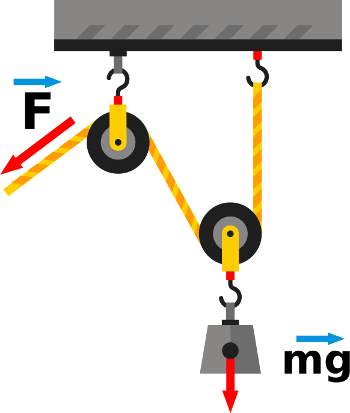# The Differences between Mass and WeightMass and weight seem to be interchangeable whenever they’re discussed, yet they’re anything but. So what is mass? What is weight? How do they differ and why is it important to know the difference? How are they linked? We’ll answer these questions and more in this blog post. Please keep in mind this explanation is simplified.

## What is mass?

Mass is a measure of how much matter an object is composed of. Usually, mass is measured in grams or kilograms. Here’s a fun fact: in the imperial system, mass is not measured in ounces or pounds, but with a unit called the slug. Mass is linked to Force, as it can be measured by determining the amount of Force it takes to change the direction or speed of an object. You’re probably familiar with this equation: F (force) = m (mass) times a (acceleration). Mass is also linked to gravity, which is basically the attraction between two objects that have mass depending on distance. However, they are not related; gravity has no effect on mass.Mass is also indestructible (like energy, and it’s a scalar as well), and can never be equal to zero (think about it: mass is the measure how much of matter is in an object. How can an object be composed of zero matter?).

## What is weight?

Here’s something you might not have known: the metric unit for weight is the Newton, not the gram. The imperial unit for weight is the pound. Remember mass and gravity being linked? Weight is the gravitational interaction between objects that have mass. If you’ve looked at the differences between mass and weight before, you’ve probably seen something about your mass on Earth and the Moon being the same, but you’ll weigh less on the Moon than you do on Earth because the Moon’s mass is not the same as Earth’s (so its gravity is not the same). Weight can be equal to zero (depending on the gravity) and is force (and therefore a vector).## Why the Confusion?

Well, for one, mass and weight are not only related, but proportional. If you know something’s mass, you can find out its weight, and vice versa, using the formula W (weight) = m (mass) times g (gravity, on Earth usually valued at 9.8 N/kg). Since we live on Earth and usually don’t have to consider mass on another planet (unless you’re a scientist), we usually use these terms somewhat interchangeably. Furthermore, weight being expressed in Newtons would be confusing to most people. If I say my cat weighs 10 pounds (or 4.5 kilos), you know he’s about average, but if I say he’s about 44 Newtons, that’s meaningless to most people.

## Scales, Balances, Mass and Weight

Scales give you the weight of something – to be technical, they measure how much force is exerted on the load cell (weight is measured in Newtons, remember?) - and then uses gravity to give you your mass. Other scales (like TBBs) measure mass by comparing the known mass and the unknown mass.## Why it’s Important not to Confuse them

Mass is a key variable in a lot of different formulas for basic concepts. It must be exact, or else it can throw everything into disarray. Gravity and even altitude can affect weight, and even the slightest miscalculation could be disastrous or completely wrong in fields like aerospace, astronomy, physics, chemistry and more. Because mass is a fundamental property, it shapes our understanding of the world and how it works, and how we can affect it.